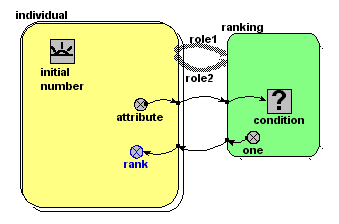# Model entry

Assigning rank order to the instances of a population submodel - Model catalogue - Simulistics.com

 Search Simulistics Model catalogue Listed by keyword Listed by ID Listed by title Listed by date added

# Assigning rank order to the instances of a population submodel

Model : ranking1
Simile version : 3.1+
Keywords : Technique ; Ranking ; Population submodel ;

## Description

There are a number of situations where you might want to rank the members (instances) of a population submodel in order, according to some criterion.

For example, you might want to select the three fattest pigs to send off to market. One way to do this is to define an association between pairs of instances, which holds true when the first member of the pair has a greater value for some attribute than the second. Then, the fattest pig will have no instances of this association (since there are no pigs with a greater value for fatness than itself). If there are (say) eleven pigs in the population at some point in time, then the middle pig will have five instances of this association, while the smallest pig will have ten instances. All that you then need to do is to count up, for each pig, the number of instances of this association that it is linked to, and use this rank value to kill off the individual.

This example model shows the bare bones of the solution. We have a population submodel, and an association submodel. The attribute in the population submodel used for ranking is (in this case) simply a random value. The condition for the association submodel simply tests whether the value for the attribute through role 1 is greater than through role 2. The association submodel has a variable ('one'), whose value is set to 1 - and we then just add this up (and add 1 to the result) to give the rank of each instance of the population submodel.

## Diagram## Equations

```Equations in submodel 'individual'
attribute = rand_const(0,100)
rank = count({one_role2})+1
where: {one_role1} = ../ranking/one (to individual in role1)
creation:    initial number = 10

Equations in submodel 'ranking'
condition = (attribute_role1>attribute_role2)
where:
attribute_role1 = ../individual/attribute (from individual in role1)
attribute_role2 = ../individual/attribute (from individual in role2)]
one = 1
```